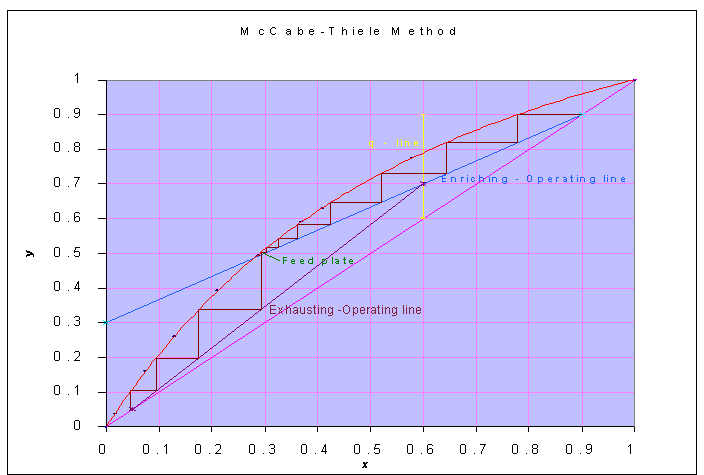### McCabe Thiele Method

A saturated liquid mixture containing 60 mole % benzene and 40 mole % toluene is to be distilled continuously into a distillate product containing 90 mole % benzene and the bottom product containing 5 mole % benzene. The fractional distillation column will operate at approximately constant pressure of 1 atm. The reflux ratio is 2. How many theoretical plates must the column have if the feed is introduced into the eighth plate? Equilibrium data are:

 x 0 0.017 0.075 0.13 0.211 0.288 0.37 0.411 0.581 0.78 1 y 0 0.039 0.161 0.261 0.393 0.496 0.591 0.632 0.777 0.9 1

Data:

zF = 0.6

xD = 0.9

xW = 0.05

R = 2

xD/(R + 1) = 0.9/(2 + 1) = 0.3

Feed is saturated liquid

Calculations:

For saturated liquid feed, slope of 'q-line' is ∞.

The equilibrium data are plotted and by McCabe-Thiele method, number of theoretical plates is found to be = 11 for feed introduced at 8th plate.